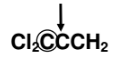Problem: What is the total number of valence electrons that should be shown explicitly as bonding pairs and/or lone pairs in the correct Lewis structure for Cl2CCCH2? A. 24        B. 25       C. 26       D. 27       E. 28 Look at the carbon atom marked with the arrow in the condensed structural formula for Cl2CCCH2 that is given in the question above. What is the geometrical arrangement of electron groups surrounding this carbon atom in Cl2CCCH2? A. Trigonal planar B. Tetrahedral C. Linear

FREE Expert Solution
100% (92 ratings)
Problem Details

What is the total number of valence electrons that should be shown explicitly as bonding pairs and/or lone pairs in the correct Lewis structure for Cl2CCCH2?

A. 24        B. 25       C. 26       D. 27       E. 28

Look at the carbon atom marked with the arrow in the condensed structural formula for Cl2CCCH2 that is given in the question above. What is the geometrical arrangement of electron groups surrounding this carbon atom in Cl2CCCH2?

A. Trigonal planar

B. Tetrahedral

C. Linear